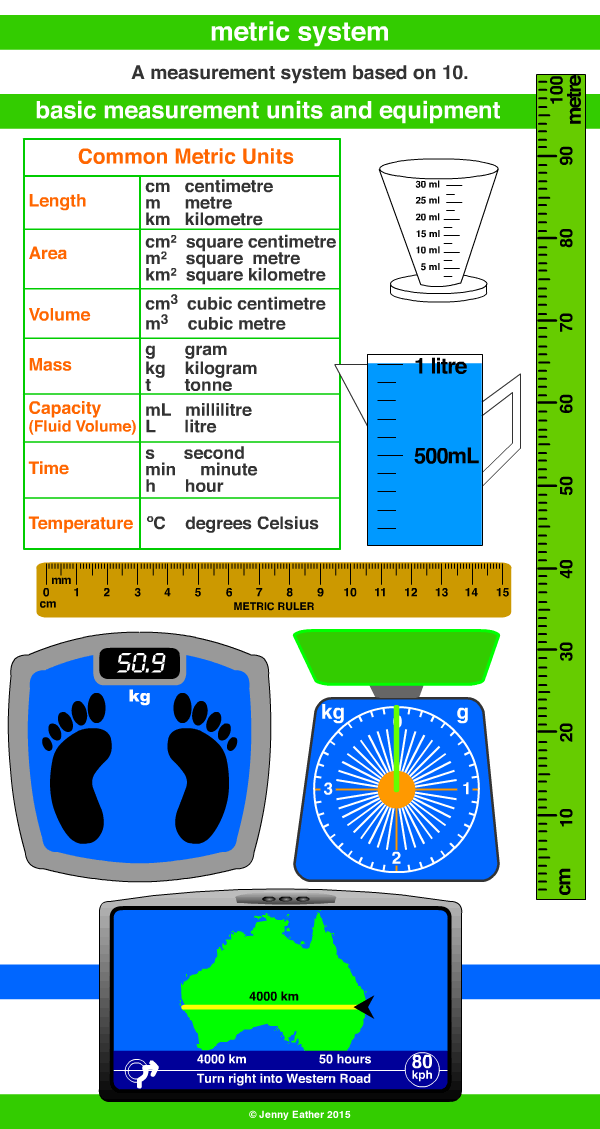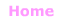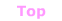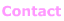Aa Bb Cc Dd Ee Ff Gg Hh Ii Jj Kk Ll Mm Nn Oo Pp Qq Rr Ss Tt Uu Vv Ww Xx Yy Zz

Mm

metric system

• a decimal system of measurement based on 10.
• officially called the International System of Units or SI
which is the modern form of the metric system and
the most widely used system in the world.
• the S.I. base units are mass - the kilogram,
time - the second, length - the metre.
• all other units are derived from these.

EXAMPLES:Spelling Note: US conventions -re becomes -er

Metric Units ~ Length

10 millimetres = 1 centimetre, cm
10 centimetres = 1 decimetre, dm
10 decimetres = 1 metre, m
10 metres = 1 decametre, Dm or dkm
10 decametres = 1 hectometre, hm
10 hectometres = 1 kilometre, km
1000 metres = 1 kilometre, km

Metric Units ~ Area

1 square metre = 10 000 square centimetres, cm2
1 hectare = 10 000 square metres, m2
1 square kilometre = 100 hectares, ha

Metric Units ~ Mass

1000 milligrams = 1 gram, g
1000 grams = 1 kilogram, kg
1000 kilograms = 1 tonne, t

Metric Units ~ Volume

1000 cubic millimetres = 1 cubic centimetre, cm3
1000 cubic centimetres = 1 cubic decimetre, dm3
1,000 cubic decimetres = 1 cubic metre, m3
1,000,000 cubic centimetres = 1 cubic metre, m3

Metric Units ~ Capacity/Fluid Volume

10 millilitres = 1 centilitre, cl or cL
10 centilitres = 1 decilitre, dl or dL
10 decilitres = 1 litre, l or L
1000 litres = 1 kilolitre, kl or kL
1,000,000 litres = 1 megalitre, Ml or ML
1,000,000,000 litres = 1 gigalitre, Gl or GL
1,000,000,000,000 litres = cubic kilometre = 1 teralitre, Tl or TL

Metric Units ~ Temperature

Celsius, ºC also known as Centigrade, ºC© Jenny Eather 2014. All rights reserved.